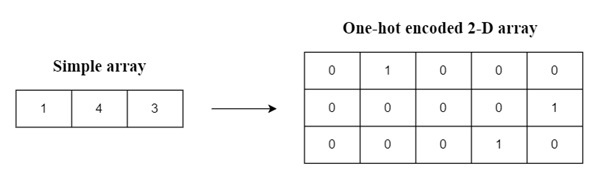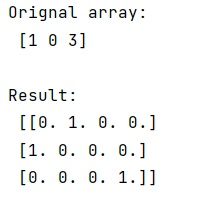# Convert array of indices to one-hot encoded array in NumPy?

In this tutorial, we will learn how to convert array of indices to one-hot encoded array in NumPy? By Pranit Sharma Last updated : May 24, 2023

NumPy is an abbreviated form of Numerical Python. It is used for different types of scientific operations in python. Numpy is a vast library in python which is used for almost every kind of scientific or mathematical operation. It is itself an array which is a collection of various methods and functions for processing the arrays.

## Converting array of indices to one-hot encoded array in NumPy

One hot encoding is a technique used in machine learning algorithms to convert categorical data like 1/0, yes/no, true/false, etc into binary values of 0 and 1.

Numpy arrays can be indexed based on the indices of the elements. Here, a NumPy array is converted into a one-hot encode two-dimensional array. We can understand this process with the help of this pictorial representation.A two-dimensional array is created whose number of rows is equal to the size of the array and the number of columns is equal to the largest element of the original array added to 1.

Let us understand with the help of an example,

## Python program to convert array of indices to one-hot encoded array in NumPy

```# Import numpy
import numpy as np

# Creating a numpy array using full method
arr = np.array([1, 0, 3])

# Display original array
print("Orignal array:\n",arr,"\n")

# Creating a one hot encode array
res = np.zeros((arr.size, arr.max() + 1))
res[np.arange(arr.size), arr] = 1

# Display result
print("Result:\n",res)
```

### Output